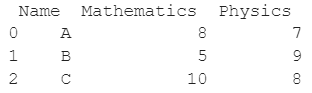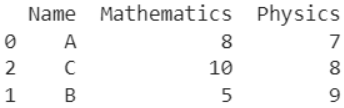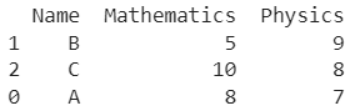# How To Sort A Pandas DataFrame Based On Values From One Column?

A DataFrame is the primary data structure of the Pandas library and is commonly used for storing and working with tabular data.

A common operation that could be performed on such data is to sort the rows of the DataFrame in an ascending or descending order based on the values of a particular column in order to add more meaning to the information.

To start working with Pandas, we first need to import it in Python code:

``import pandas as pd``

## Running Example

Let us understand this operation with the help of an example. Consider the following DataFrame containing 3 students with names A, B, and C and their corresponding marks (out of 10) for two subjects, Mathematics and Physics.Code snippet for generating the above DataFrame :

### Python 3 Code :

``````import pandas as pd

# Dictionary for our data
data = {'Name' : ['A', 'B', 'C'], 'Mathematics' : [8, 5, 10], 'Physics' : [7, 9, 8]}

# DataFrame for the dictionary
df = pd.DataFrame(data)

# Printing the DataFrame
print(df)``````

Here, data is a dictionary we created to initialize the DataFrame. For this, we use the DataFrame() function of the Pandas library which takes the dictionary as an argument and returns the required DataFrame.

Now let us say that for some reason, we want to sort the rows of this DataFrame based on the values in a given column, say Physics. If we sort in ascending order, the resulting output will look as follows:If we perform the sorting operation in descending order, the resulting DataFrame will look as follows :Let us look at different ways of performing this operation on a given DataFrame :

## Sorting Dataframe Using sort_values() function - ascending order and in place

In this method, we use the DataFrame.sort_values() function to sort the rows based on the values in a particular column of a given Pandas DataFrame.

Let the column of interest be the one with the label as Physics here. The DataFrame.sort_values() function takes the first parameter as the label of the column based on which sorting needs to be done.

The parameter inplace is set to True to specify that the sorting needs to be done in an inplace manner so that no reassignment is required.

The third parameter ascending is set to True to specify that sorting needs to be done in ascending order.

Note that the original row index is preserved.

Let us look at the code and corresponding output for this method.

``````import pandas as pd

# Dictionary for our data
data = {'Name' : ['A', 'B', 'C'], 'Mathematics' : [8, 5, 10], 'Physics' : [7, 9, 8]}

# DataFrame for the dictionary
df = pd.DataFrame(data)

# Sort the DataFrame rows based on the values in the column labeled as Physics
df.sort_values('Physics', inplace = True, ascending = True)

# Print the new DataFrame
print(df)``````

Output :## Sorting Dataframe Using DataFrame.sort_values() function - ascending order and not in place

In this method, we use the DataFrame.sort_values() function to sort the rows based on the values in a particular column of a given Pandas DataFrame.

Let the column of interest be the one with the label as Physics here. The DataFrame.sort_values() function takes the first parameter as the label of the column based on which sorting needs to be done.

The parameter inplace is set to False to specify that the sorting does need to be done in an in place manner so reassignment is required.

The parameter ascending is set to True to specify that sorting needs to be done in ascending order. Note that the original row index is preserved.

Let us look at the code and corresponding output for this method.

``````import pandas as pd

# Dictionary for our data
data = {'Name' : ['A', 'B', 'C'], 'Mathematics' : [8, 5, 10], 'Physics' : [7, 9, 8]}

# DataFrame for the dictionary
df = pd.DataFrame(data)

# Sort the DataFrame rows based on the values in the column labeled as Physics
df = df.sort_values('Physics', inplace = False, ascending = True)

# Print the new DataFrame
print(df)``````

Output :## Sorting Dataframe Using DataFrame.sort_values() function - descending order and in place

In this method, we use the DataFrame.sort_values() function to sort the rows based on the values in a particular column of a given Pandas DataFrame.

Let the column of interest be the one with the label as Physics here. The DataFrame.sort_values() function takes the first parameter as the label of the column based on which sorting needs to be done.

The parameter inplace is set to True to specify that the sorting needs to be done in an in place manner so that no reassignment is required.

The parameter ascending is set to False to specify that sorting needs to be done in descending order. Note that the original row index is preserved.

Let us look at the code and corresponding output for this method.

``````import pandas as pd

# Dictionary for our data
data = {'Name' : ['A', 'B', 'C'], 'Mathematics' : [8, 5, 10], 'Physics' : [7, 9, 8]}

# DataFrame for the dictionary
df = pd.DataFrame(data)

# Sort the DataFrame rows based on the values in the column labeled as Physics
df.sort_values('Physics', inplace = True, ascending = False)

# Print the new DataFrame
print(df)``````

Output :## Sorting Dataframe Using DataFrame.sort_values() function - descending order and not in place

In this method, we use the DataFrame.sort_values() function to sort the rows based on the values in a particular column of a given Pandas DataFrame.

Let the column of interest be the one with the label as Physics here. The DataFrame.sort_values() function takes the first parameter as the label of the column based on which sorting needs to be done.

The parameter inplace is set to False to specify that the sorting does need to be done in an in place manner so reassignment is required.

The parameter ascending is set to False to specify that sorting needs to be done in descending order. Note that the original row index is preserved.

Let us look at the code and corresponding output for this method.

### Python 3 Code :

``````import pandas as pd

# Dictionary for our data
data = {'Name' : ['A', 'B', 'C'], 'Mathematics' : [8, 5, 10], 'Physics' : [7, 9, 8]}

# DataFrame for the dictionary
df = pd.DataFrame(data)

# Sort the DataFrame rows based on the values in the column labeled as Physics
df = df.sort_values('Physics', inplace = False, ascending = False)

# Print the new DataFrame
print(df)``````

Output :## Conclusion

In this topic, we have learned to sort the rows of the DataFrame in an ascending or descending order based on the values of a particular column of an existing DataFrame, following a running example of test scores of students in different subjects, thus giving us an intuition of how this concept could be applied in real-world situations. Feel free to reach out to info.javaexercise@gmail.com in case of any suggestions.

Trending